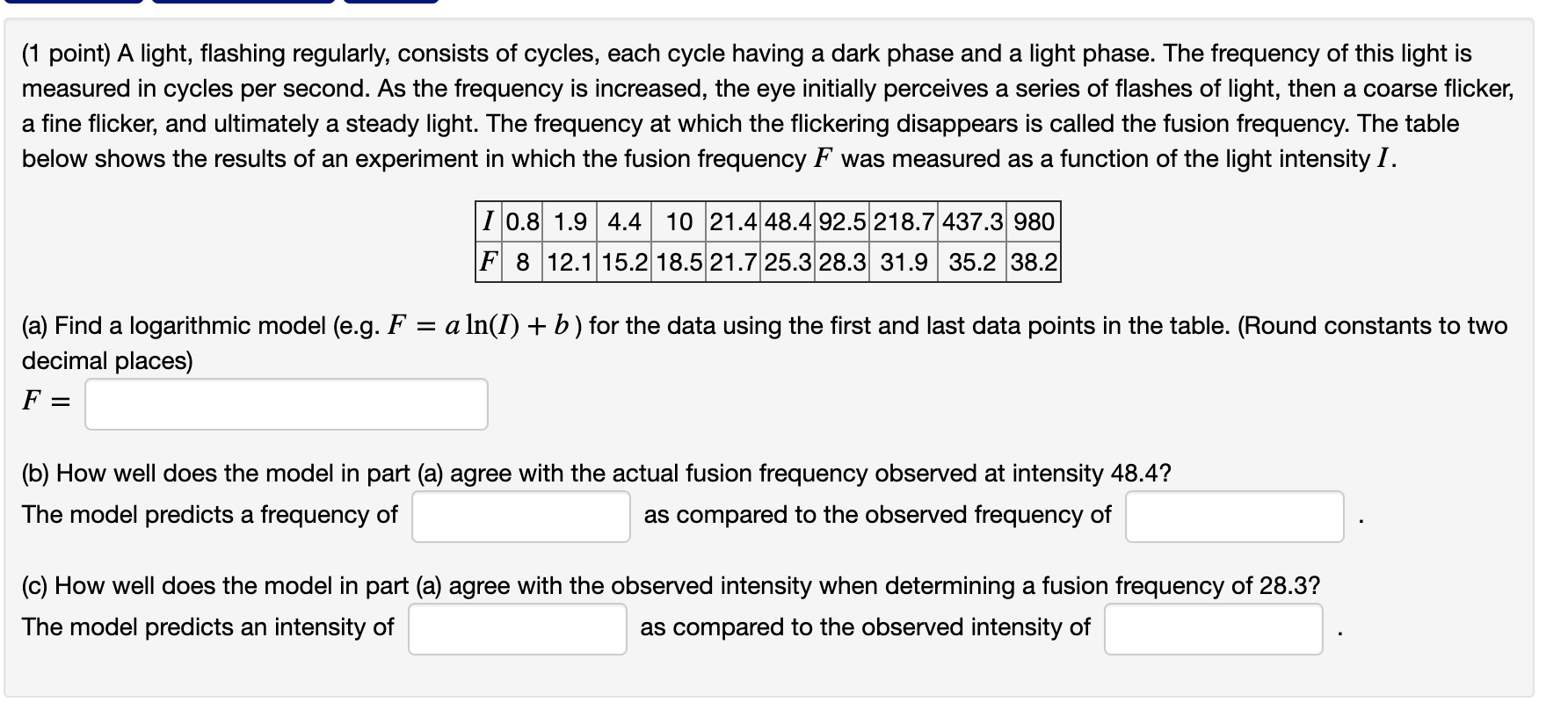# Question A light, flashing regularly, consists ofcycles, each cycle having a dark phase and a light phase. Thefrequency of this light is measured in cycles per second. As thefrequency is increased, the eye initially perceives a series offlashes of light, then a coarse flicker, a fine flicker, andultimately a steady light. The frequency at which the flickeringdisappears is called the fusion frequency. The table below showsthe results of an experiment in which the fusion frequency F wasmeasured as a function of the light intensity (1 point) A light, flashing regularly, consists of cycles, each cycle having a dark phase and a light phase. The frequency of this light is measured in cycles per second. As the frequency is increased, the eye initially perceives a series of flashes of light, then a coarse flicker, a fine flicker, and ultimately a steady light. The frequency at which the flickering disappears is called the fusion frequency. The table below shows the results of an experiment in which the fusion frequency F was measured as a function of the light intensity I. I 0.8 1.9 4.4 10 21.4 48.4 92.5 218.7 437.3 980 F 8 12.1 15.2 18.5 21.7 25.3 28.3 31.9 35.2 38.2 (a) Find a logarithmic model (e.g. F = a ln(I) + b) for the data using the first and last data points in the table. (Round constants to two decimal places) F (b) How well does the model in part (a) agree with the actual fusion frequency observed at intensity 48.4? The model predicts a frequency of as compared to the observed frequency of (c) How well does the model in part (a) agree with the observed intensity when determining a fusion frequency of 28.3? The model predicts an intensity of as compared to the observed intensity ofA light, flashing regularly, consists of cycles, each cycle having a dark phase and a light phase. The frequency of this light is measured in cycles per second. As the frequency is increased, the eye initially perceives a series of flashes of light, then a coarse flicker, a fine flicker, and ultimately a steady light. The frequency at which the flickering disappears is called the fusion frequency. The table below shows the results of an experiment in which the fusion frequency F was measured as a function of the light intensity